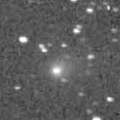# \$B%7%e!<%a!<%+!<(B-\$B%l%t%#Bh#4WB@1(B

118P/Shoemaker-Levy 4 (1997)

 English version Home page Updated on July 26, 2009###\$B%W%m%U%#!<%k(B

 \$BId9f(B 118P/1995 M1 \$B8!=PF|(B 1995\$BG/(B6\$B7n(B22\$BF|(B \$B8!=P8wEY(B 21.9\$BEy(B \$B8!=P J. V. Scotti (Kitt Peak)

###\$B###\$B50F;MWAG(B

```   The following improved orbital elements by Kenji Muraoka, are
from 237 observations 1991 to 1998, perturbations by 9 Planets,
Moon and 5 minor planets were taken into account.
The mean residual is +/- 0.75 arc seconds.

Epoch  =  1997 Feb.  1.0  TT       JDT = 2450480.5
T  =  1997 Jan. 12.11225       +/- 0.00025 (m.e.) TT
Peri. =  301.97973                +/- 0.00013
Node  =  152.09627                +/- 0.00012   (2000.0)
Incl. =    8.47342                +/- 0.00001
q  =    2.0211344              +/- 0.0000009 AU
e  =    0.4205115              +/- 0.0000002
a  =    3.4877906              +/- 0.0000006 AU
n  =    0.15131378             +/- 0.00000004
P  =    6.514                  +/- 0.0000016  years
```

###\$B@1?^(B1996\$BG/(B 6\$B7n(B 6\$BF|!A(B1997\$BG/(B 7\$B7n(B 1\$BF|(B

###\$B8wEYJQ2=(B

```        m1 =  8.2 + 5 log\$B&\$(B + 18.0 log r  [    ,-100]  (              \$B!A(B1996\$BG/(B10\$B7n(B 4\$BF|(B)
m1 = -6.0 + 5 log\$B&\$(B + 60.0 log r  [-100, 100]  (1996\$BG/(B10\$B7n(B 4\$BF|!A(B1997\$BG/(B 4\$B7n(B22\$BF|(B)
m1 =  8.2 + 5 log\$B&\$(B + 18.0 log r  [ 100,    ]  (1997\$BG/(B 4\$B7n(B22\$BF|!A(B              )

\$B"((B \$B3%?'\$N6J@~\$O!"(Bm1 = 8.2 + 5 log\$B&\$(B + 18.0 log r \$B\$N8wEY6J@~\$G\$9!#(B
```##### \$B50F;MWAG\$OB<2,7r<#;a\$N7W;;\$K\$h\$k\$b\$N\$G\$9!#(B \$B@1?^\$O(B StellaNavigator Ver.2.0 for Windows (\$B%"%9%H%m%"!<%D(B \$BJTCx(B / \$B%"%9%-!<=PHG6I4)(B) \$B\$G:n@.\$7\$?\$b\$N\$G\$9!#(B \$B8wEY%0%i%U\$O(BComet for Windows\$B\$G:n@.\$7\$?\$b\$N\$G\$9!#(B